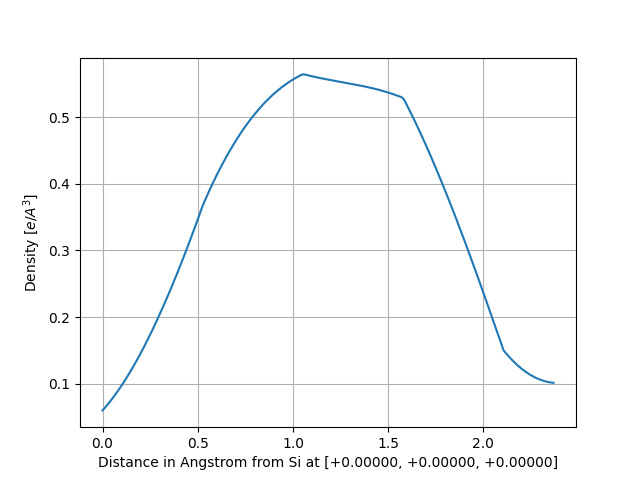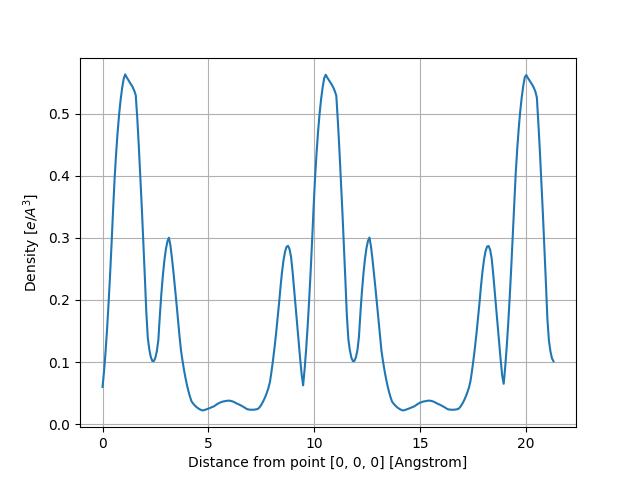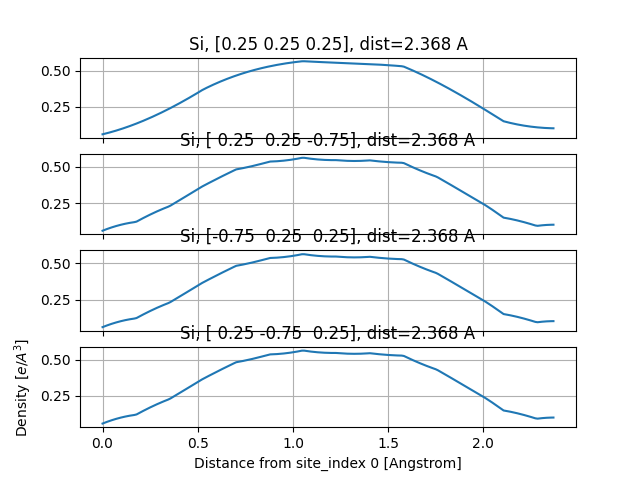# Density¶

This example shows how to analyze the electronic density stored in the DEN.nc file.

```from abipy.abilab import abiopen
import abipy.data as abidata

# Open the DEN.nc file
ncfile = abiopen(abidata.ref_file("si_DEN.nc"))

# The DEN file has a `Density`, a `Structure` and an `ElectronBands` object
print(ncfile.structure)
```

Out:

```Full Formula (Si2)
Reduced Formula: Si
abc   :   3.866975   3.866975   3.866975
angles:  60.000000  60.000000  60.000000
Sites (2)
#  SP       a     b     c
---  ----  ----  ----  ----
0  Si    0     0     0
1  Si    0.25  0.25  0.25

Abinit Spacegroup: spgid: 227, num_spatial_symmetries: 48, has_timerev: True, symmorphic: True
```

To plot the KS eigenvalues.

```ncfile.ebands.plot()

density = ncfile.density
print(density)

# To visualize the total charge wih vesta
#visu = density.visualize("vesta"); visu()
```Out:

```Density: nspinor: 1, nsppol: 1, nspden: 1
Mesh3D: nx=18, ny=18, nz=18
```

To plot the density along the line connecting the first and the second in the structure:

```density.plot_line(point1=0, point2=1)
```Out:

```<Figure size 640x480 with 1 Axes>
```

alternatively, one can define the line in terms of two points in fractional coordinates:

```density.plot_line(point1=[0, 0, 0], point2=[2.25, 2.25, 2.25], num=300)
```Out:

```<Figure size 640x480 with 1 Axes>
```

To plot the density along the lines connect the firt atom in the structure and all the neighbors within a sphere of radius 3 Angstrom:

```density.plot_line_neighbors(site_index=0, radius=3)
```Out:

```<Figure size 640x480 with 4 Axes>
```

Total running time of the script: ( 0 minutes 0.693 seconds)

Gallery generated by Sphinx-Gallery